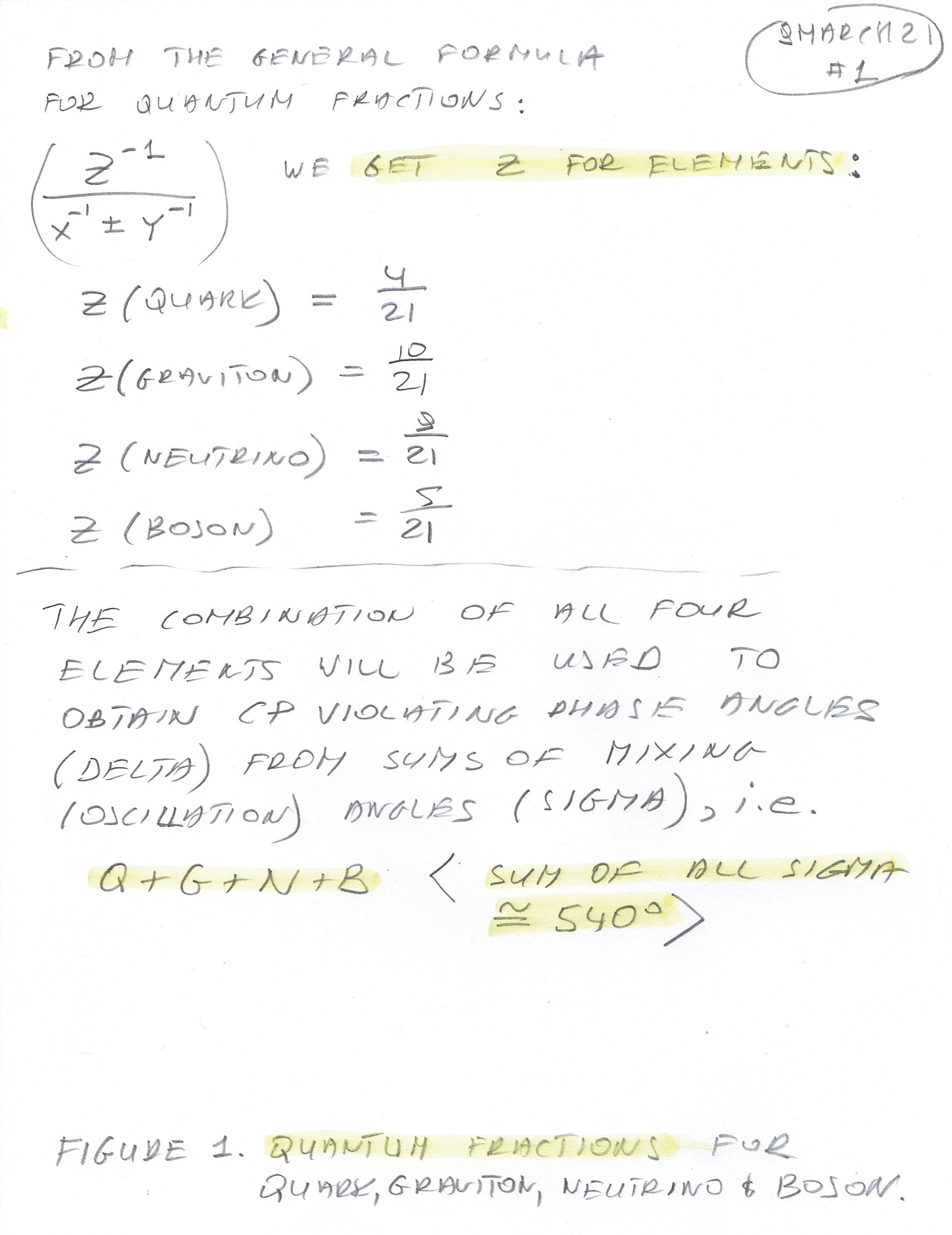Forty Holy Martyrs of Sebaste, Armenia (320); St Macarius (334)

In this article, I will show how to obtain CP Violating Phase Angles (Delta) from sums (Sigma) of the Mixing (Oscillation) Angles (Theta) for Quark, Graviton, Neutrino and Boson using Quantum Fractions.

All we need is the sums (Sigma) of the Mixing (Oscillation) Angles (Theta) for all four elements (Quark, Graviton, Neutrino and Boson) and their respective Quantum Fractions. Also, we will need Spherical Correction Angles for Quark and Graviton - those were calculated before.

In "Figure 2 - Sums of Mixing (Oscillation) Angles Theta, for Quark, Graviton, Neutrino and Boson" those angles (Sigma) are highlighted in yellow. Just to recall - Sums (Sigma) are the sums of all three mixing (oscillation) angles Theta (from experiments) and their values after the Transform into the Positive Real part of the Complex Plane.

Sigma = Theta Initial + Theta Final (after the Transform)

Here is Figure 2 - Sums of Mixing (Oscillation) Angles Theta of Quark, Graviton, Neutrino and Boson:In "Figure 1 - Quantum Fractions of Quark, Graviton, Neutrino and Boson" are listed these fractions for all four elements. Also, I have chosen from many unique ways of calculating CP Violating Phase Angles (Delta) the one which seems to be the best - calculating Delta from the sums of all four elements, i.e. (Quark + Graviton + Neutrino + Boson). These sums ( Sigma) add to approximately 540 degrees (remember, that this is spherical geometry).

Here is Figure 1 - Quantum Fractions for Quark, Graviton, Neutrino and Boson:1. Calculating exact CP Violating Phase Angle (Delta) of Quark from the sum (Sigma) of Mixing (Oscillation) Angles (Theta).

First, the Spherical Correction Angle of Quark.

Spherical Correction Angles. Those are just the sums of the angles of different elements and reduced to multiplications of 90+ deg (or if you prefer multiplications of 22.5+ deg).

Correction Angles for combinations of the elements:

( Quark + Graviton ) + ( Boson + Neutrino ) = ( 1 / 3 ) x + ( 2 / 3 ) y = 90.289527 deg

The above angle times 2 has to be deducted from the sum of all four Sigmas as follows (I do not know yet why):

Quadruplet (the sum of the angles = 540 deg i.e. Pentagon shape):

Quark + Graviton + Boson + Neutrino = (90.4706 deg + 90.5494 deg +102.8371 deg + 257.8801 deg - 2 X 90.28953 deg) X ( 4 / 21 ) = 361.15811 deg X ( 4 / 21 ) = δCP VIOLATION PHASE ANGLE QUARK  = 68.79202 deg = 1.20064725 radians

For comparison, here is the official (from 2008) value of Delta CP Violating Phase Angle of Quark:

Delta Quark = 68.7549 deg(+/- 4.58 degrees)

If you want the details, the original article is here:

75. Revisited: The Exact value of the CP Violation Phase Angle of Quark.

2. Calculating exact CP Violating Phase Angle (Delta) of Graviton from the sum (Sigma) of Mixing (Oscillation) Angles (Theta).

First - the Spherical Correction Angle of Graviton is the same as for Quark:

Spherical Correction Angles. Those are just the sums of the angles of different elements and reduced to multiplications of 90+ deg (or if you prefer multiplications of 22.5+ deg).

Correction Angles for combinations of the elements:

( Quark + Graviton ) + ( Boson + Neutrino ) = ( 1 / 3 ) x + ( 2 / 3 ) y = 90.289527 deg

The above angle times 2 has to be deducted from the sum of all four Sigmas as follows (I do not know yet why):

Quadruplet (the sum of the angles = 540 deg i.e. Pentagon shape):

Quark + Graviton + Boson + Neutrino = (90.4706 deg + 90.5494 deg +102.8371 deg + 257.8801 deg - 2 X 90.28953 deg) X ( 10 / 21 ) = 361.15811 deg X ( 10 / 21 ) = δCP VIOLATION PHASE ANGLE GRAVITON  = 171.98005 deg = 3.00161813 radians

If you want the details, the original article is here:

80. The Exact value of the CP Violation Phase Angle of Graviton.

3. Calculating exact CP Violating Phase Angle (Delta) of Neutrino from the sum (Sigma) of Mixing (Oscillation) Angles (Theta).

The Spherical Correction Angle not needed in this case.

Quadruplet (the sum of the angles = 540 degrees i.e. Pentagon shape):

Quark + Graviton + Boson + Neutrino = δ CP VIOLATION PHASE ANGLE NEUTRINO = (90.4706 deg + 90.5494 deg +102.8371 deg + 257.8801 deg + 0 X 90+ deg) X ( 9 / 21 ) = 541.73716 deg X ( 9 / 21 ) = 232.1731 deg = 4.05218448 radians

For comparison, here is the official value of Delta CP Violating Phase Angle of Neutrino:

Delta CP Violating Phase Angle of Neutrino from Experiments:

Delta Neutrino = 234 degrees (+ 43 deg; -31 deg) (year 2018)

If you want the details, the original article is here:

4. Calculating exact CP Violating Phase Angle (Delta) of Boson from the sum (Sigma) of Mixing (Oscillation) Angles (Theta).

The Spherical Correction Angle not needed in this case.

Quadruplet (Pentagon - the sum of internal angles equal to 540 degrees):

Quark + Graviton + Boson + Neutrino = δ CP VIOLATION PHASE ANGLE BOSON  = ( 90.4706 + 90.5494 + 102.8371 + 257.8801 ) x ( 5 / 21 ) = 128.985038 deg = 2.25121360 radians

If you want the details, the original article is here:

70. Exact value of the CP Violation Phase Angle of Boson.

As you can see, these values of CP Violating Phase Angles are looking good and in the case of Quark and Neutrino, they agree perfectly with experimental data (there is no experimental data yet for Boson and Graviton).

In the next series of articles, I will derive sums (Sigma) of Mixing (Oscillation) Angles and CP Violating Phase Angles (Delta) for the remaining four elements of Quantum World/Universe, i.e. Space, Time, Membrane Internal and Membrane External.